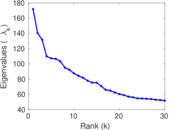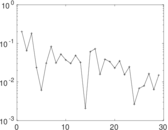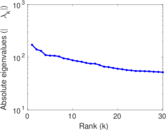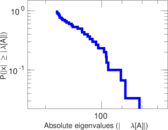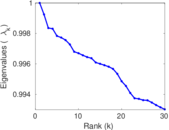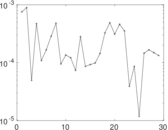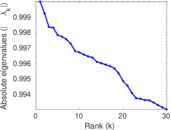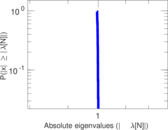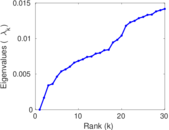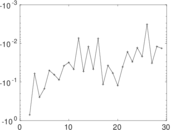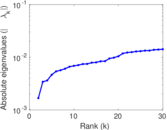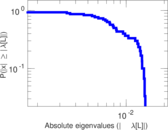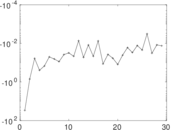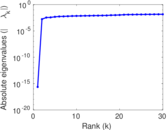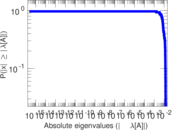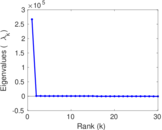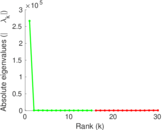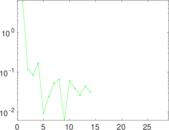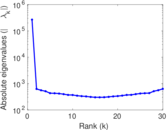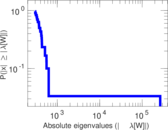# Genres (DBpedia)

This is the bipartite genre network from DBpedia. The nodes in the network are works and artists, as well as genres from music, film and other areas. Each edge connects a work or artist to a genre. The edges correspond to the <http://dbpedia.org/ontology/genre> relationships in DBpedia.

 Code `GE` Internal name `dbpedia-genre` Name Genres (DBpedia) Data source http://wiki.dbpedia.org/Downloads AvailabilityDataset is available for download Consistency checkDataset passed all tests Category Feature network Dataset timestamp 2001 ⋯ 2017 Node meaning Work, genre Edge meaning Style Network formatBipartite, undirected Edge typeUnweighted, no multiple edges

## Statistics

 Size n = 266,717 Left size n1 = 258,934 Right size n2 = 7,783 Volume m = 463,497 Wedge count s = 1,331,590,538 Claw count z = 6,667,061,771,791 Cross count x = 32,482,545,125,451,776 Square count q = 54,299,350 4-Tour count T4 = 5,761,771,762 Maximum degree dmax = 24,821 Maximum left degree d1max = 31 Maximum right degree d2max = 24,821 Average degree d = 3.475 57 Average left degree d1 = 1.790 02 Average right degree d2 = 59.552 5 Fill p = 0.000 229 991 Size of LCC N = 259,138 Diameter δ = 32 50-Percentile effective diameter δ0.5 = 3.706 53 90-Percentile effective diameter δ0.9 = 7.435 10 Median distance δM = 4 Mean distance δm = 5.076 13 Gini coefficient G = 0.645 943 Balanced inequality ratio P = 0.258 995 Left balanced inequality ratio P1 = 0.377 167 Right balanced inequality ratio P2 = 0.073 191 4 Relative edge distribution entropy Her = 0.773 524 Power law exponent γ = 3.236 04 Tail power law exponent γt = 1.621 00 Tail power law exponent with p γ3 = 1.621 00 p-value p = 0.000 00 Left tail power law exponent with p γ3,1 = 5.651 00 Left p-value p1 = 0.214 000 Right tail power law exponent with p γ3,2 = 1.541 00 Right p-value p2 = 0.000 00 Degree assortativity ρ = −0.078 141 0 Degree assortativity p-value pρ = 0.000 00 Spectral norm α = 171.978 Algebraic connectivity a = 0.001 674 73 Spectral separation |λ1[A] / λ2[A]| = 1.223 27 Controllability C = 251,772 Relative controllability Cr = 0.944 551

## Plots

### Fruchterman–Reingold graph drawing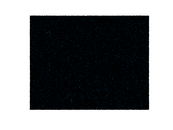### Degree distribution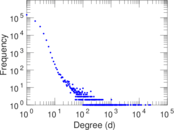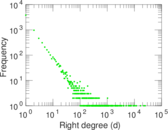### Cumulative degree distribution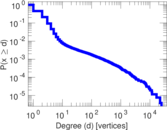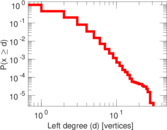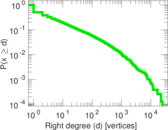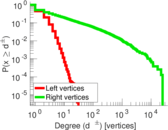### Lorenz curve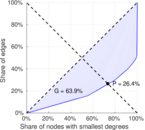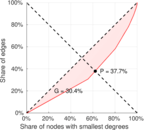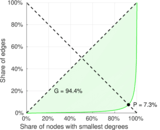### Spectral distribution of the adjacency matrix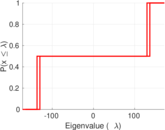### Spectral distribution of the normalized adjacency matrix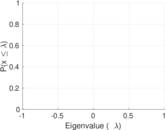### Spectral distribution of the Laplacian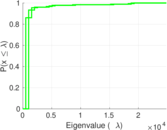### Spectral graph drawing based on the adjacency matrix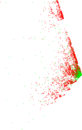### Spectral graph drawing based on the Laplacian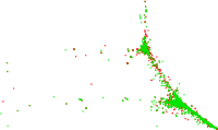### Spectral graph drawing based on the normalized adjacency matrix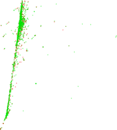### Degree assortativity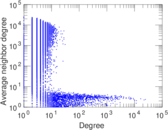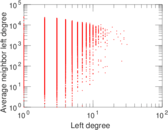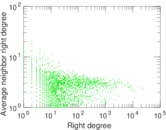### Zipf plot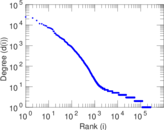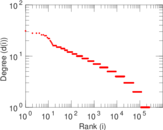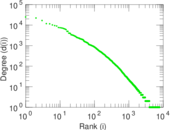### Hop distribution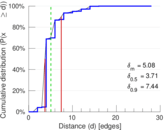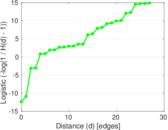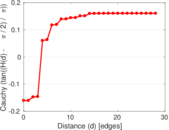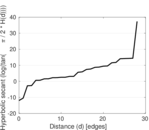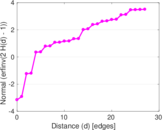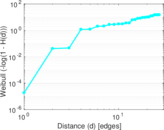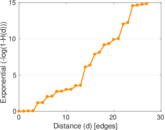### Delaunay graph drawing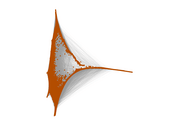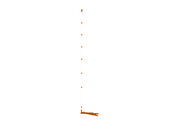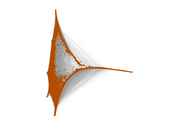### Matrix decompositions plots